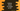# Python program to check if a series is Geometric progression## Python program to check if a series is Geometric progression:

In this post, we will learn how to check if a series is geometric progression or not in Python. This program will take one array or series of numbers as input and print one message if that series is Geometric progression/GP or not.

### Algorithm to use:

Geometric progression is a series of numbers where each number is calulated by multiplying the previous number by a constant value. This value is called common ratio.

So, if we divide any number by its previous number, it will be a constant value.

We can write one program as like below to find out if a series is GP or not:

• Find out the ratio of second number/ first number. Store that value in a variable.

• Run one loop from the third number to the end of the list. For each number, divide it by its preceding number and compare it with the ratio calculated on previous step.
• If it is not equal, return False.
• Once the loop ends, return True. Because all rations are equal. So, this is a Geometric progression.

## Python program to check for GP:

Below is the complete python program:

``````def check_geometric_progression(arr):
l = len(arr)

common_ratio = arr/arr

for i in range(2, l):
if arr[i]/arr[i - 1] != common_ratio:
return False

return True

first_list = [4, 12, 36, 108, 324]
second_list = [2, 4, 8, 16, 32, 69]

print(check_geometric_progression(first_list))
print(check_geometric_progression(second_list))``````

### Explanation:

Here,

• checkgeometricprogression method is used to check if an array is Geometric progression or not. It returns one boolean value. True if it is a GP, else False.
• common_ratio is the ratio of second element/first element. We will compare this value with other elements of this series.
• The for loop runs from the third element to end of the list.

• The if statement checks if the ratio of current element/previous element is equal to common_ratio or not. If not, it returns False.
• Once the for loop ends, it returns True.

### Output:

If you run the above program, it will print the below output:

``````True
False``````